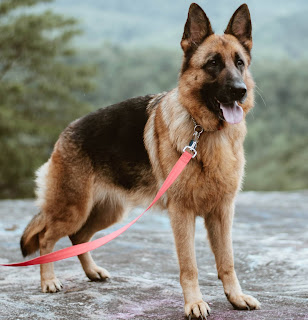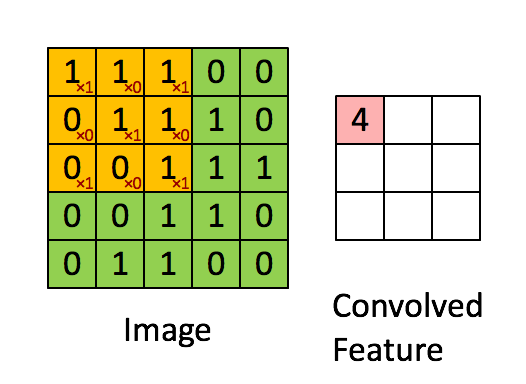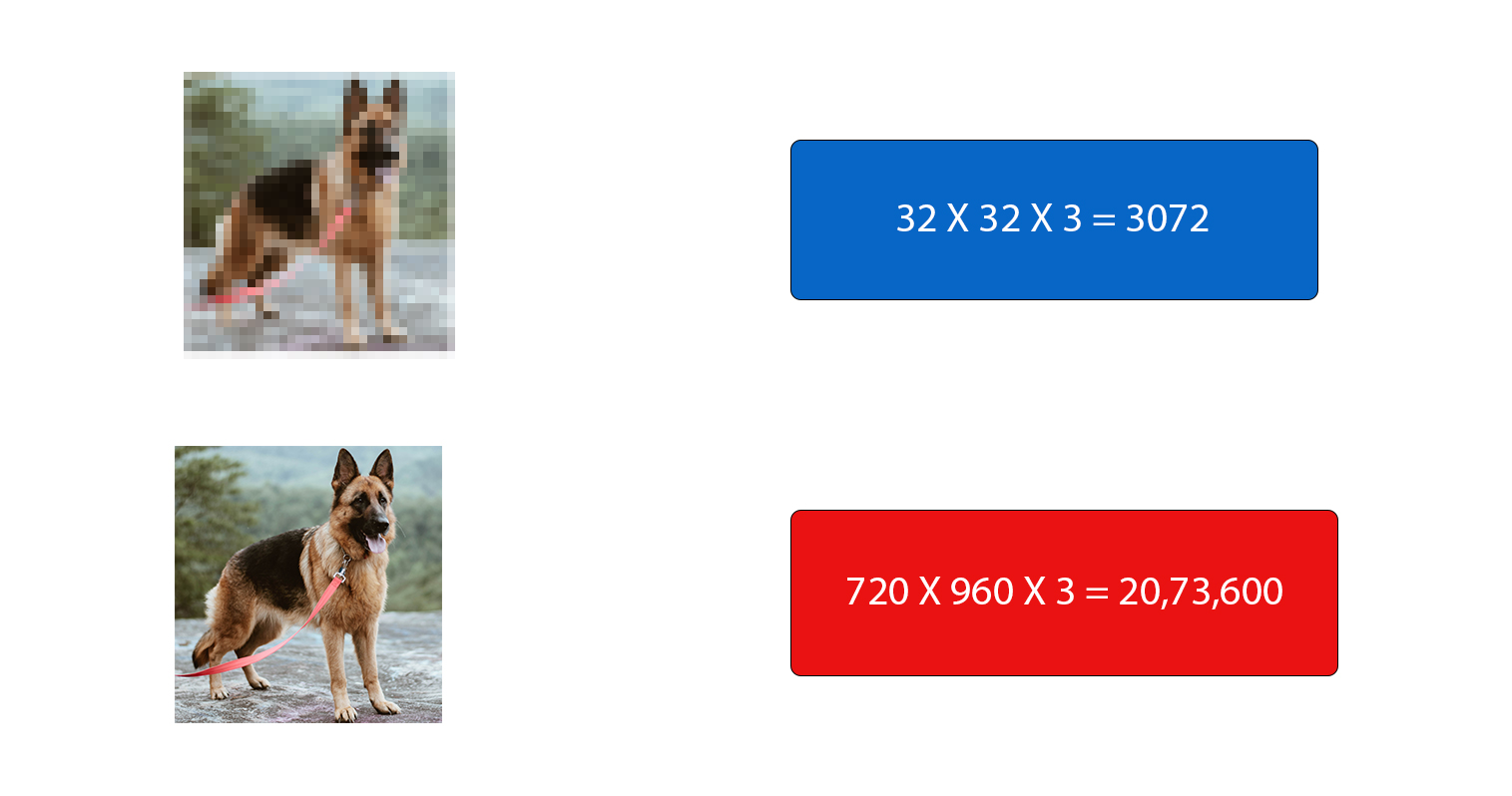Convolutional Neural Networks are easier than you thinkPhoto by Kristopher Roller on Unsplash

In our last article on neural networks, we learned how neural networks work. In this article we will look into another type of a neural network used in deep learning, Convolution Neural Network also known as ConvNet/CNN.

What is Convolutional neural network?

A convolutional neural network is a Deep Learning algorithm which is designed for working with two dimensional images. It applies a filter to the input image to extract the features from the input. The same filter is applied multiple times to the input to generate a feature map which indicates the strength of the detected features. The pre processing required in a ConvNet is much lower as compared to other classification algorithms.

Why CNN? Why not use simple Neural Network?

To answer this question first we need to understand how convNet works.

How convolutional neural networks works?

Before understanding the working of convolutional neural networks let us understand how we humans identify an object in the image.

Let’s take this image of a dog. How do we identify that it’s a dog in the image?Original dog Photo by Katelyn MacMillan

We start by looking at its ears, tail, nose, eyes and in our brain different neurons are working on these features and then these neurons transfer this information to another neurons which combine all these features to come up to a result that if the image has dog’s ear, eyes, nose then there is dog’s face in the image. Similarly, if there are dog’s legs and tail in the image then there is dog’s body in the image. Again, these features are combined and our brain come to a result that if there is dog’s face and body in the image then it’s a dog’s image.

So, now how can we make computer recognize these tiny features? We use the concept of filters. We pass a filter to the image and that filter moves across the pixels of the image and gives an output with the detected feature. The process of moving of this filter across the pixels of the image is known as convolution or convolution operation and this is what “Convolution” represents in CNN. If you are wondering how this filter moves across the image then take a look at the below animation you will get an idea how it works.Convolution operation

So, coming back to our question, why CNN in place of simple neural networks? what is the problem with Fully connected neural networks?

The first problem with fully connected neural networks is that they lose spatial orientation of the content in the images. Didn’t get it? Don’t worry, look at images below.

Original Dog Photo by Anna Dudkova

Can you identify the difference between 1 and 2? no, right? Now look at image 3 and 4. The 3rd one is the normal image of a dog while the 4th one is the manipulated image where one of dog’s eye is replaced with nose.  Looking at the 2D or 3D form makes it very easy to identify the abnormalities in the images but in 1D form it is very difficult to identify the abnormalities in the images. A dog is a dog only when eyes, ears, nose are relatively present where they should be. CNN preserves the spatial orientation by using filters, it takes a 2d or 3d input and works to create features which do not lose spatial orientation until necessary.

Another problem with the fully connected neural networks is with the computation. Look at the two images below.Original dog Photo by Katelyn MacMillan

The first image is of size 32x32x3, so if we have one hidden layer containing just 1000 neurons then the number of parameters comes around 3 million. The second image is of size 720X960X3 and with 1000 neurons in the hidden layer the numbers of parameters explode to around 2 billion this will be a nightmare for any computer system. Now, imagine a condition where you might want to get 3 to 4 layers deep with each layer containing 1000 neurons. This problem is known as parameter explosion. CNN use pooling for dimensionality reduction. It uses local connectivity instead of full connectivity.

We will discuss about filters and pooling layers in another article. For now, let’s look at the basic architecture of the CNN.

CNN Architecture

•  Input layer – Takes image input.
•  CNN - Performs feature extraction.
• Fully connected neural network - Combines the features extracted by CNN to reach an output.CNN architecture

Watch this video and learn how pooling layer works in CNN.

Understanding mean Average Precision for Object Detection (with Python Code)

Photo by  Avel Chuklanov  on  Unsplash If you ever worked on object detection problem where you need to predict the bounding box coordinates of the objects, you may have come across the term mAP (mean average precision). mAP is a metric used for evaluating object detectors. As the name suggest it is the average of the AP. To understand mAP , first we need to understand what is precision, recall and IoU(Intersection over union). Almost everyone is familiar with first two terms, in case you don’t know these terms I am here to help you. Precision and Recall Precision: It tells us how accurate is our predictions or proportion of data points that our model says relevant are actually relevant. Formula for precision Recall: It is ability of a model to find all the data points of inte